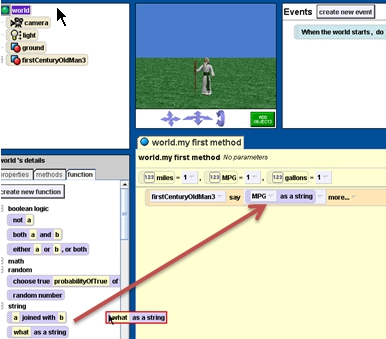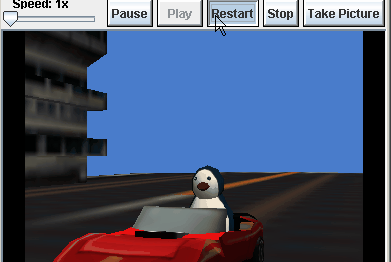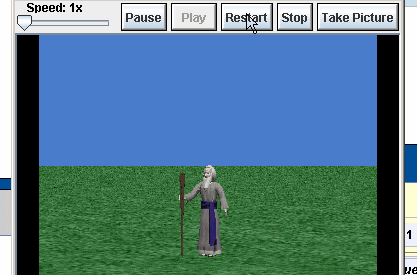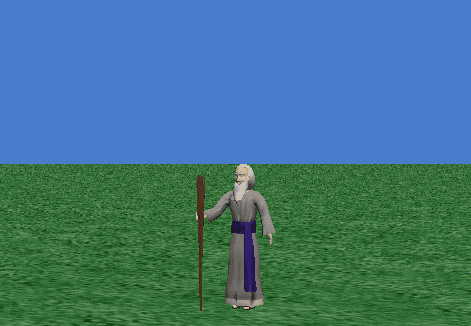# Unit 4** Alice Assignments

Assignment ): Tutorial 4-2 Gum Drop Fish Assignment will be graded (2 points) from packet #13 will be graded.  Download the GumdropBigFish world

Assignment 1 )   Miles Per Gallon: Create a world in which the

How to use “what as a string”Assignment  3) Fahrenheit to Centigrade :

First, watch the animated gifs on this web page that explain how to use math in Alice

• Create a variable called degreesFahrenheit  .
• Store the return value of the ask_user to enter a number function
• You should ask them for the degrees in Fahrenheit
• Create a variable called  degreesCentigrade
• Use the following formula to convert the degreesFahrenheit   to Centigrade using the following formula
• use a character from the`People `collection to say the centigrade temperature.
• Don’t forget that you can’t say numbers, you must use what as a stringAssignment 4) Modification of MPG

reopen the miles per gallon project

• if the MPG variable <  20
• say “What a Gas Guzzler”
• else
• say ” Your car is not a gas guzzler”Assignment 5) Modification of Fahrenheit to Centigrade

• if the degreesCentigrade  variable <30
• say “It’s Very cold
• else
• say ” It is warm outside”

Assignment 6) Circle’s Area and Circumference: Create a world with your choice of person from the `People`collection. When you play the world, it should

• ask the user for the radius of a circle. (This value should be stored in a variable ).
• The person should then say the area and the circumference of the circle.( Links for the circumference formula , area of a circle)
• (For an extra point do the same thing with a “Sphere“. Ask the user for the radius and calculate the surface area and the volume.

Assignment 8Roman Numeral translator

Here’s a gif showing partial code and what the finished project should look like
Objective : to let the user type in a number between 1 and 10 and then have a character “translate” that into Roman Numerals ( I, II, III, IV, V, VI, VII, VIII, IX, X)

We are going to use the roman Numeral Translator file again, so it’s important that you don’t lose the file and give it a good name that you can easily recognize in the future.

Assignment 7-extra credit ). Look up the formulas for the volume and surface area of a cylinder . Ask the user for the height of the cylinder and the radius, which should be stored in variables. Then calculate the surface area and volume of the cylinder . See if there is a cylinder shape in the gallery to use as a visual aid.

Assignment 8 ) Open Assignment

Create an Alice world that

• uses a variable
• uses a ask_user world function
• uses a comment
• uses an if/else
• uses a math expression with more than one operation (ie addition and also multiplication or multiplication and also subtraction)
• tells a story

Part I